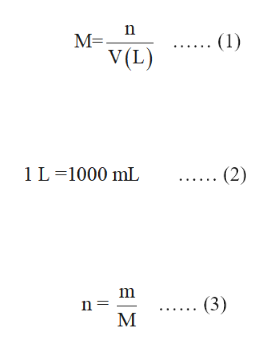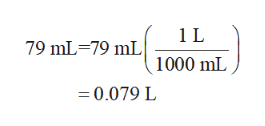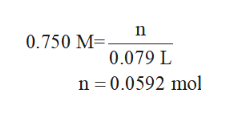How many grams of Ag2CO3 will precipitate when excess Na2CO3 solution is added to 79.0 mL of 0.750 M AgNO3 solution

Question

How many grams of Ag2CO3 will precipitate when excess Na2CO3 solution is added to 79.0 mL of 0.750 M AgNO3 solution

Step 1

The molarity of a solution is the ratio of number of moles of a substance to the volume of solution in liters. The expression for molarity is labelled in equation (1) in which M is the molarity, n is the number of moles of solute and  V is the volume in liters.

The relation between millilitres (mL) and liters (L) is written in equation(2).

The number of moles of a substance is the ratio of its given mass by molar mass of the substance. It is expressed in equation (3) in which n is the number of moles, m is the given mass and M is the molar mass.help_outlineImage TranscriptioncloseМ-. V(L) (1) .. (2) 1 L 1000 mL (3) n = E Z l fullscreen
Step 2

Convert 79.0 mL in L by using the equation (2) as shown below.help_outlineImage Transcriptionclose1 L 79 mL 79 mL 1000 mL =0.079 L fullscreen
Step 3

The reaction of sodium carbonate and silver nitrate is written below.

2AgNO3(aq) + Na2CO3(aq) → Ag2CO3 (s) +2NaNO3(aq)

The number of moles of sil...help_outlineImage Transcriptionclosen 0.750 M= 0.079 L n 0.0592 mol fullscreen

Want to see the full answer?

See Solution

Want to see this answer and more?

Our solutions are written by experts, many with advanced degrees, and available 24/7

See Solution
Tagged in

Chemistry